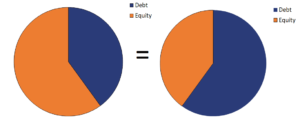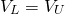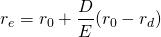# Capital Structure Irrelevance Proposition

The Capital Structure Irrelevance Proposition shows that, under a very restrictive set of assumptions, the value of a firm is unaffected by its capital structure. As such, it does not matter how a firm finances its operations. The capital irrelevance theory was proposed by Merton Miller and Franco Modigliani in 1958. The theory implies that there is no such thing as an optimal capital structure.

On this page, we discuss the assumptions underlying the capital irrelevance proposition and we provide the formula that generates the weighted average cost of capital (WACC) under these assumptions.

## Capital structure irrelevance assumptions

The assumptions that are required for the capital structure to be irrelevant are the following:

1. No agency costs: no costs from increased leverage
2. Investment decisions are unaffected by financing decisions: revenues from operations are independent of how the operations are financed
3. Riskless borrowing and and lending
4. Investors have the same expectations on the company’s prospects
5. Capital markets are perfectly competitive: no transaction costs, taxes, or bankruptcy costs

Under these assumptions, the capital structure is irrelevant. The following graph illustrates this concept. No matter how the pie is composed, the size (value) of the pie is the same.Or, expressed using a formula, we getwhere VL is the value of the leveraged firm and VU is the value of the unleveraged firm.

## Capital structure irrelevance formula

There is also a second proposition that states that the cost of equity will increase linearly with the proportion of debt financing. That’s because, as the proportion of debt goes up, the equity becomes riskier because debtholders have a priority claim. The larger proportion of cheaper debt offsets the increase in the cost of equity. Thus, the WACC remains constant.

The following formula captures this relationshipwhere re is the required rate of return on equity, r0 is the company’s unlevered cost of equity, rd is the required rate of return on debt, and D/E is the debt-to-equity ratio.

## Summary

We discussed Miller and Modigliani’s (1958) Proposition I and II. In the absence of taxes, the WACC is constant and the capital structure does not influence the value of the firm.